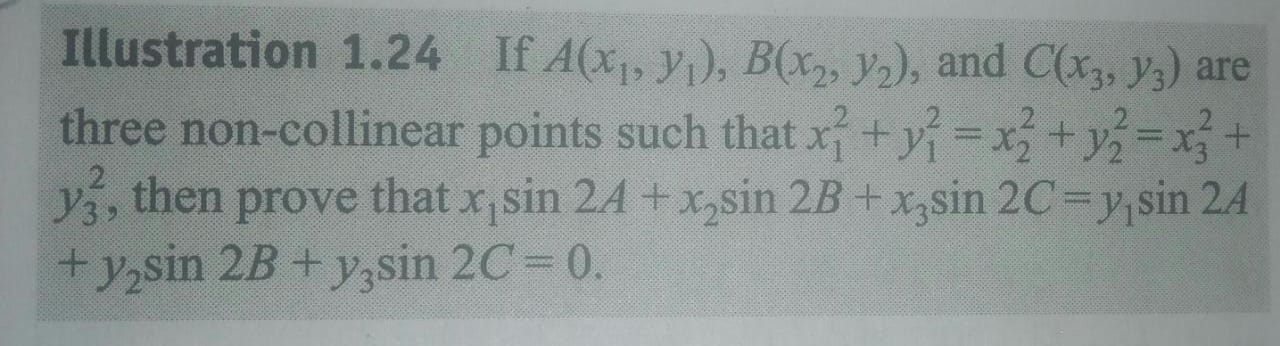# Doubt from Coordinate GeometryX1²+Y1² is nothing but distance of point X1 , Y1 from origin
And it is given that distance of three points from the origin are same which implies that the circumcentre of these 3 point is 0,0 and hence
x coordinate of circumcentre = y coordinate of circumcentre = 0
And Thus we can say
{(x1 sin 2A + x2 sin 2B + x3 sin 2C)/ (sin 2A + sin 2B + sin 2C)=0=(y1 sin 2A + y2 sin 2B + y3 sin 2C)/ (sin 2A + sin 2B + sin 2C)}.

OR
(x1 sin 2A + x2 sin 2B + x3 sin 2C)=0=(y1 sin 2A + y2 sin 2B + y3 sin 2C)

1 Like

Thanks sir

1 Like